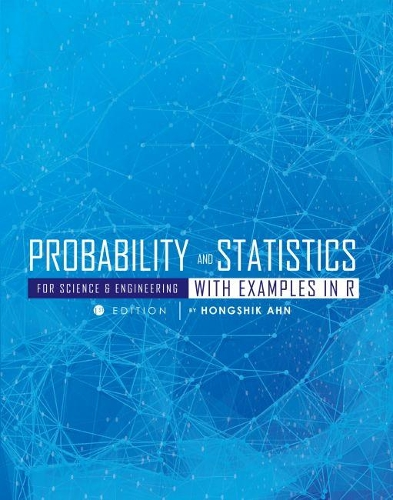•Probability and Statistics for Science and Engineering with Examples in R (Paperback)

(author)
£82.50
Paperback 342 Pages / Published: 30/01/2017
• Not available

This product is currently unavailable.

Probability and Statistics for Science and Engineering with Examples in R teaches students how to use R software to obtain summary statistics, calculate probabilities and quantiles, find confidence intervals, and conduct statistical testing.

The first chapter introduces methods for describing statistics. Over the course of the subsequent eight chapters students will learn about probability, discrete and continuous distributions, multiple random variables, point estimation and testing, and inferences based on one and two samples. The book features a comprehensive table for each type of test to help students choose appropriate statistical tests and confidence intervals.

Based on years of classroom experience and extensively class-tested, Probability and Statistics for Science and Engineering with Examples in R is designed for one-semester courses in probability and statistics, and specifically for students in the natural sciences or engineering. The material is also suitable for business and economics students who have studied calculus.

Publisher: Cognella, Inc
ISBN: 9781516513987
Number of pages: 342
Dimensions: 229 x 152 mm